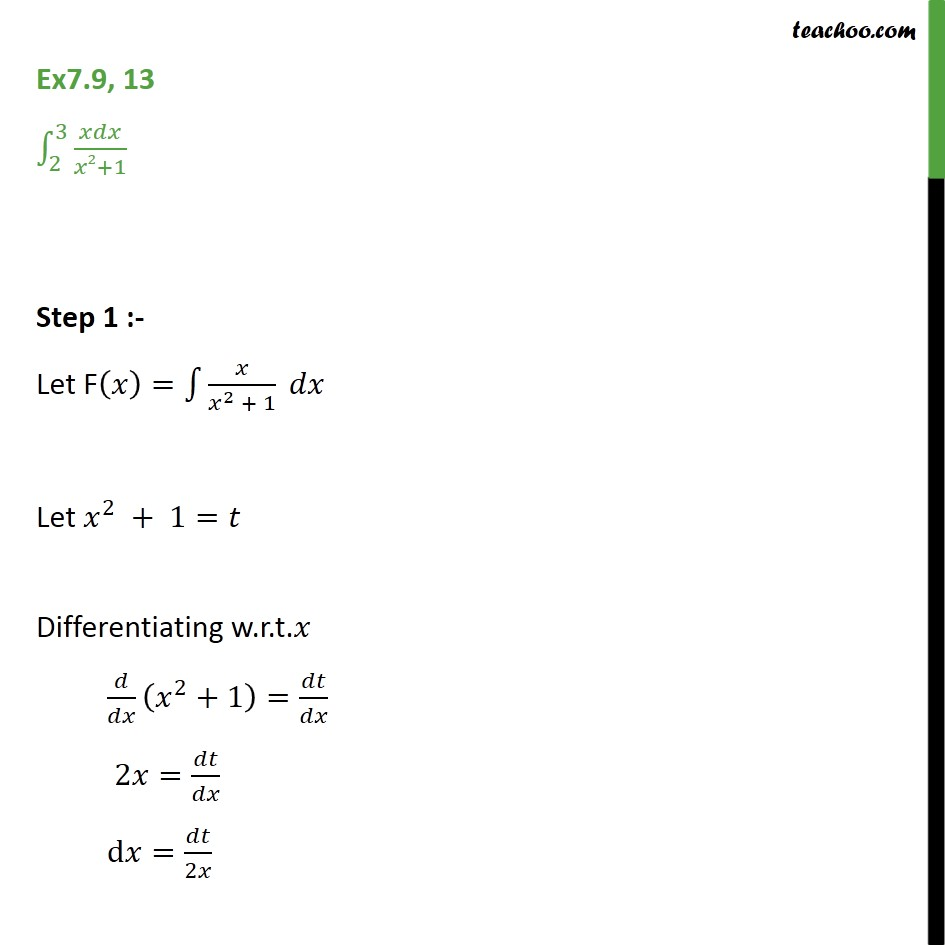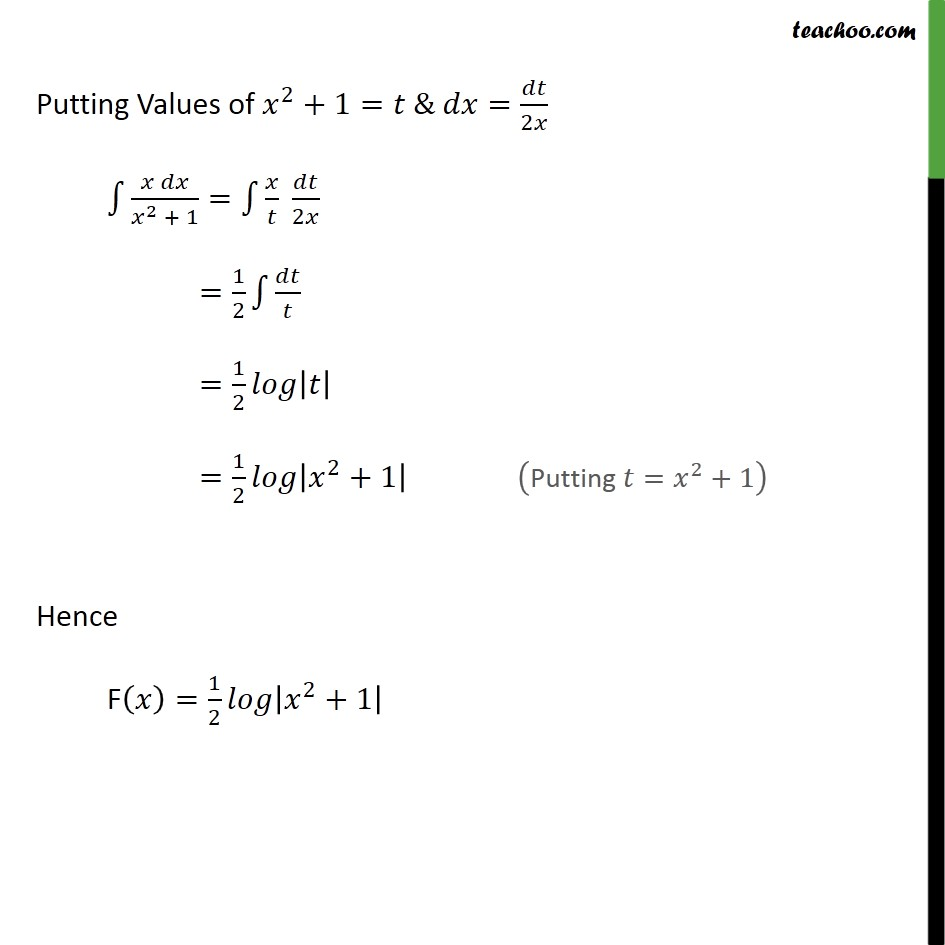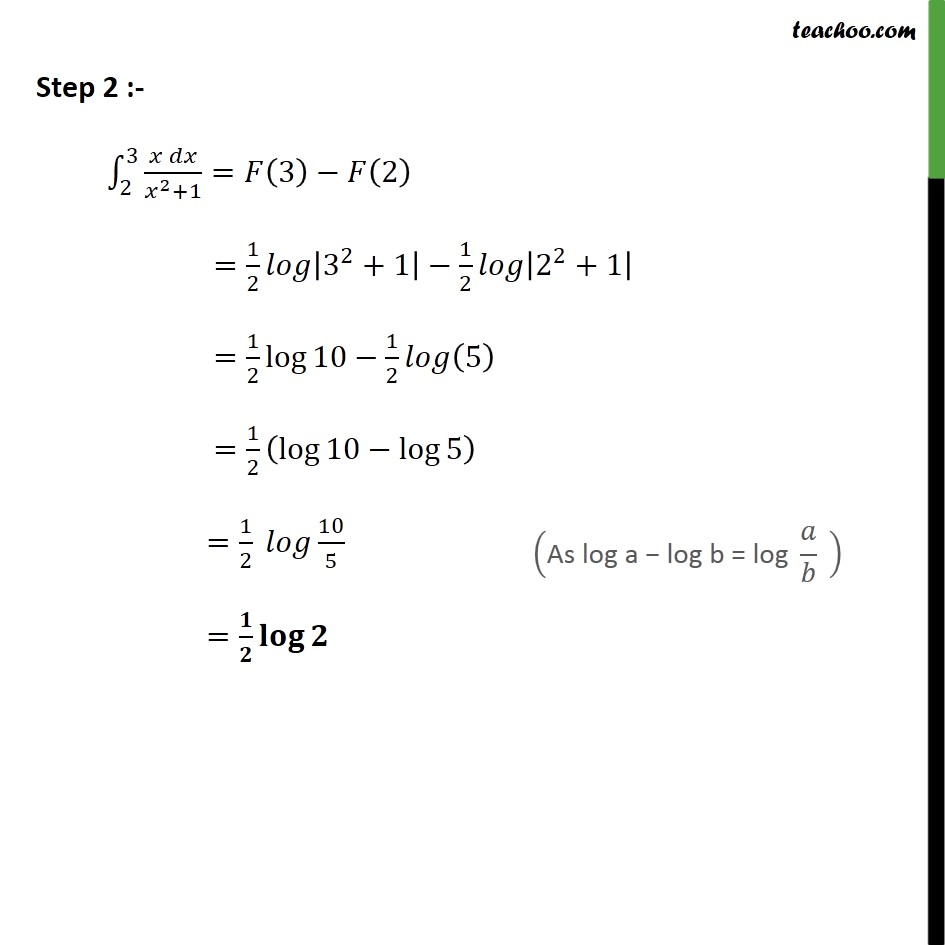Definite Integration - By Substitution

Chapter 7 Class 12 Integrals
Concept wiseIntroducing your new favourite teacher - Teachoo Black, at only ₹83 per month

### Transcript

Ex7.9, 13 2 3 2+1 Step 1 :- Let F = 2 + 1 Let 2 + 1= Differentiating w.r.t. 2 +1 = 2 = d = 2 Putting Values of 2 +1= & = 2 2 + 1 = 2 = 1 2 = 1 2 = 1 2 2 +1 Hence F = 1 2 2 +1 Step 2 :- 2 3 2 +1 = 3 2 = 1 2 3 2 +1 1 2 2 2 +1 = 1 2 log 10 1 2 5 = 1 2 log 10 log 5 = 1 2 10 5 =